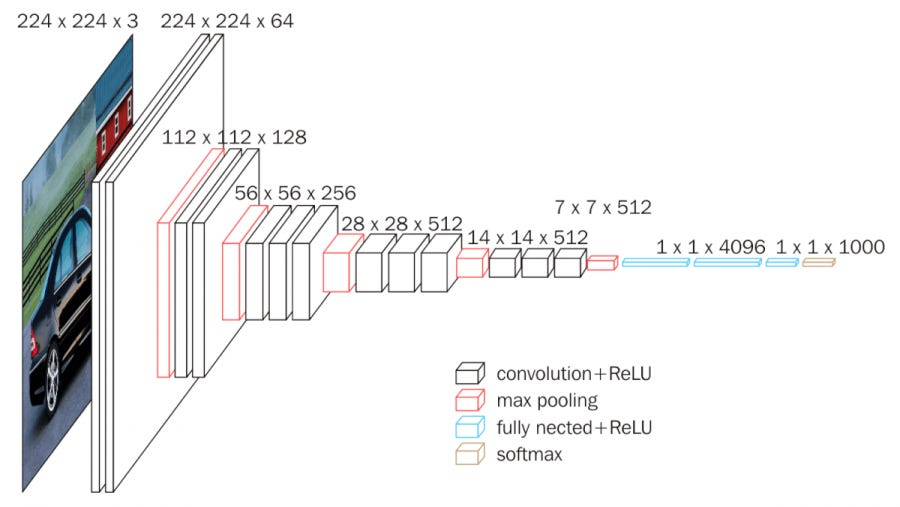# Very Deep Convolutional Networks for Large-Scale Image Recognition

Source: Deep Learning on Medium

Understanding the importance of depth in Conv. Nets.VGG-16, named after the number of conv. layers.

### Introduction

1. In this paper, an important aspect of ConvNet architecture design — its depth — is given importance. To this end, other parameters are fixed and only depth of the network is increased steadily by adding more convolutional layers, which is feasible due to the use of very small (3 × 3) convolution filters in all layers.
2. This paper is among a series of such papers aiming to improve upon AlexNet.

### Architecture

1. Input during training is a 224 by 224 by 3 image.
2. Preprocessing: Subtracting mean RGB value from each pixel.
3. Filter size was 3 by 3, the smallest that captures notions of left/right, up/down and center.
4. Stride for CONV is 1 pixel and padding is SAME.
5. Max-pooling over 2 by 2 pixel window, with stride 2. (5 times)
6. 3 Fully Connected layers, 4096|4096|1000 way softmax.
7. All hidden layers have ReLU rectification.
8. Width of conv. layers (no. of channels) starts at 64 and increases by 2 until 512 after each pooling.
9. The architectures of all configurations (from the paper):

### Discussion

1. A stack of two (3 by 3) conv. layers has an effective receptive field of (5 by 5) and 3 layers will have an effective receptive field of (7 by 7).
2. Advantage over single 7 by 7 layer: We incorporate 3 non-linear rectifications instead of 1 making the decision function more discriminative. Number of parameters decreases from 49C2 to 27C2 which is a 81% decrease. This can be looked at as imposing regularization.

### Training

1. Namely, the training was carried out by optimising the multinomial logistic regression objective using mini-batch gradient descent with momentum. The batch size was set to 256, momentum to 0.9. The training was regularised by weight decay (the L2 penalty multiplier set to 5 * 10−4 ) and dropout regularisation for the first two fully-connected layers (dropout ratio set to 0.5).
2. The learning rate was initially set to 10−2 , and then decreased by a factor of 10 when the validation set accuracy stopped improving. In total, the learning rate was decreased 3 times, and the learning was stopped after 370K iterations (74 epochs).
3. As compared to AlexNet, the nets required less epochs to converge due to (a) implicit regularisation imposed by greater depth and smaller conv. filter sizes; (b) pre-initialisation of certain layers.
4. The initialization of layers other than A were done with the first few layers of A which was already trained, this sped up the training process. However, learning rate wasn’t decreased during training of the other layers.